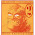## Sunday, February 03, 2008

### Scala tutorialOn a cold gray sunday afternoon, I went through the tutorial for the Scala programming language (by a group in Switzerland called LAMP). There was an exercise straight out of SICP about evaluating simple algebraic expressions. Here's what I came up with. It may be far from optimal, but seems to work for at least the one test case.

```abstract class Tree
case class Sum(l: Tree, r: Tree) extends Tree
case class Var(n: String) extends Tree
case class Const(v: int) extends Tree

object Expressions {
type Environment = String => int

def eval(t: Tree, env: Environment): int = t match {
case Sum(l, r) => eval(l, env) + eval(r, env)
case Var(n) => env(n)
case Const(v) => v
}

def derive(t: Tree, v: String): Tree = t match {
case Sum(l, r) => Sum(derive(l, v), derive(r, v))
case Var(n) if (v == n) => Const(1)
case _ => Const(0)
}

// there's probably a more functional way to do this
def simplify(t: Tree): Tree = t match {
case Sum(l, r) =>
val sl: Tree = simplify(l)
val sr: Tree = simplify(r)
sl match {
case Const(lv) => sr match {
case Const(rv) => Const(lv + rv)
case _ =>
if (lv==0) sr
else Sum(sl,sr)
}
case _ => sr match {
case Const(rv) if (rv==0) => sl
case _ => Sum(sl,sr)
}
}
case Var(n) => Var(n)
case Const(v) => Const(v)
}

def toString(t: Tree): String = t match {
case Sum(l, r) => "(" + toString(l) + " + " +
toString(r) + ")"
case Var(n) => n
case Const(v) => v.toString()
}

def main(args: Array[String]) {
val exp: Tree = Sum(
Sum(Var("x"),Var("x")),
Sum(Const(7),Var("y")))
val env: Environment = { case "x" => 5 case "y" => 7 }
println("Expression: " + toString(exp))
println("Evaluation with x=5, y=7: " + eval(exp, env))
println("Derivative relative to x: " +
toString(derive(exp, "x")) + " = " +
toString(simplify(derive(exp, "x"))))
println("Derivative relative to y: " +
toString(derive(exp, "y")) + " = " +
toString(simplify(derive(exp, "y"))))

}
```

The subclasses of Tree define the nodes of a parse tree for simple expressions like:

`((x + x) + (7 + y))`

The program demonstrates Scala's pattern matching capabilities, defining methods that perform operations on trees: evaluate, take derivatives, and print. Pattern matching is a feature borrowed from the ML family of languages. You might think of them as case statements on steroids. Sometimes they can concisely replace polymorphism. Here, they play the role of the visitor pattern.

The visitor pattern makes it easy to add new operations on all members of an inheritance hierarchy in a modular way. (Modular meaning the operation is encapsulated). The cost is that adding a new member to the inheritance hierarchy requires modification of all existing operations. The cost and benefit of pattern matching statements is the same.

More Scala Resources:

1.A probably more functional version of simplify (my first attempt at Scala):

def simplify(t: Tree): Tree = t match {
case Sum(l, r) if (l == Const(0)) => simplify(r);
case Sum(l, r) if (r == Const(0)) => simplify(l);
case Sum(l, r) if (l == Const(0) && r == Const(0)) => Const(0);
case Sum(l: Const, r: Const) => Const(l.v + r.v);
case Sum(l, r) if (simplify(l) == Const(0) || simplify(r) == Const(0)) => simplify(Sum(simplify(l), simplify(r)));
case Sum(l, r) => Sum(simplify(l), simplify(r));
case _ => t;
}

2.Thanks yardus, I like it. It didn't occur to me to let the cases fall through like that. Maybe there's a pattern matching syntax that would match the Const(0) cases, I don't know.

3.I think this also works:

def simplify(t:Tree):Tree =
t match {
case Sum(l, r) =>
(simplify(l), simplify(r)) match {
case (Const(a), Const(b)) => Const(a + b)
case _ => Sum(simplify(l), simplify(r))
}
case _ => t
}

4.Just realized it's kind of silly to simplify each subtree twice.

def simplify(t:Tree):Tree =
t match {
case Sum(l, r) =>
val sl:Tree = simplify(l)
val sr:Tree = simplify(r)
(sl, sr) match {
case (Const(a), Const(b)) => Const(a + b)
case _ => Sum(sl, sr)
}
case _ => t
}

5.This avoid simplifying the subtrees twice and also catches the cases where one side of the Sum is zero:

def simplify(t: Tree): Tree = t match {
case Sum(l, r) => {
(simplify(l), simplify(r)) match {
case (Const(0.), y) => y
case (x, Const(0.)) => x
case (Const(x), Const(y)) => Const(x + y)
case (x, y) => Sum(x, y)
}
}
case t => t
}

6.Very nice to btsai and Martin... both are much cleaner than my awkward attempt.

7.Stupid me, forgot about Const(0). I like the elegance of your code, Martin :)